## Portfolio Report

From the portfolio details page, you can access a comprehensive report revealing portfolio results.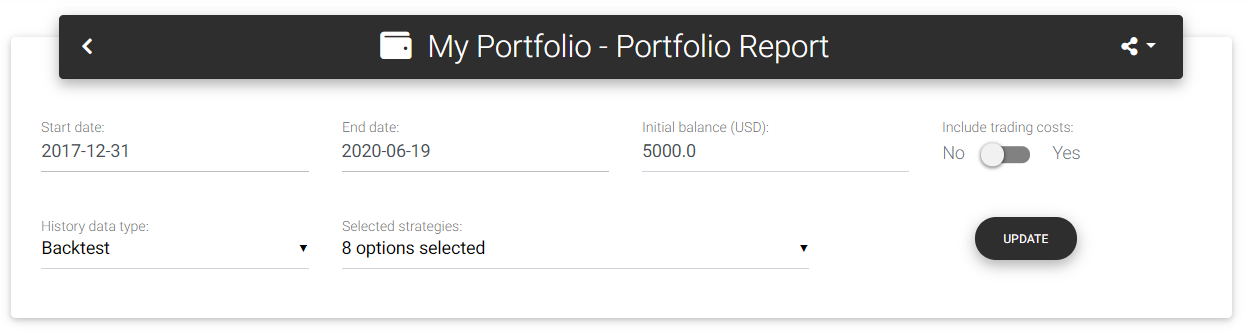Portfolio report

You can select the start and end dates to filter the history and change the starting balance used in the calculations. From the report, it is also possible to change the composition, including or excluding strategies, but keep in mind that this change will be automatically saved in the database.

By default, trading expenses are not taken into account when calculating portfolio's results. If you want to include costs in the calculation, check the option "Include trading costs."

### Main statistics

In the first part of the report, you will see the leading statistics and a chart with the equity curve. The card "Main statistics" shows the following information: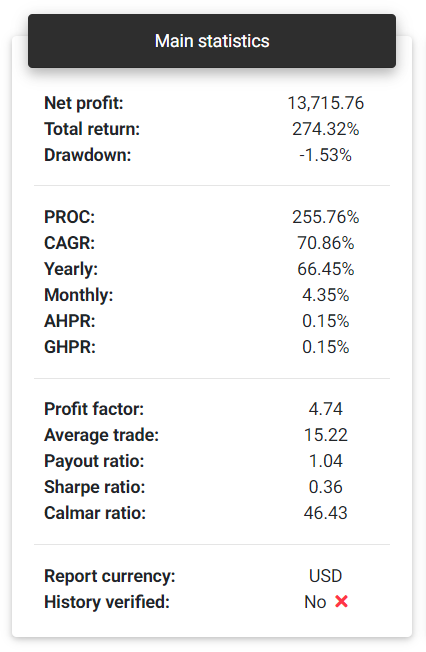Main statistics

Net profit: This is the total net profit achieved by the portfolio in the selected period. It's the sum of all individual results of the strategies that make up the portfolio.

Total return: Indicates the portfolio's percentage return, based on the net profit and initial balance.

Tip: All percentage returns are dependent on the initial balance defined for the portfolio.

Drawdown: This is the maximum percentage drawdown verified in the balance. This measure indicates how much your balance has decreased after reaching any maximum point.

PROC: Pessimistic Return on Capital is an adjusted measure of percentage return, taking into account the portfolio's win rate and average profit. This measure can give a more conservative indication of the expected return for a portfolio.

CAGR: Compound Annual Growth Rate is the annual return rate needed to obtain the final balance from the portfolio's initial balance.

Yearly: Yearly return calculated for the portfolio.

Monthly: Monthly return calculated for the portfolio.

AHPR: Average Holding Period Return is the average return of each trade, which is the average balance change for each day.

GHPR: Geometric Holding Period Return is similar to AHPR but applies a weight with the number of days with trade.

Profit factor: The profit factor is calculated as the ratio between the sum of all profits and all losses.

Average trade: Average expected result per day, that is, the average daily profit from trades.

Payout ratio: It's the ratio between the average profit and the average loss. It gives an insight into the portfolio's risk-reward ratio.

Sharpe ratio: It's the ratio of the portfolio's net return (compared to a risk-free rate) and the standard deviation of these returns. To simplify the calculations, in this case, the risk-free rate is considered zero.

Calmar ratio: This metric can also be called the Drawdown ratio. It's the ratio between the average annual return and the maximum drawdown of the portfolio.

Report currency: Shows the currency used in the report, defined in the portfolio configuration. The app converts all strategies data to this currency.

History verified: Indicates whether strategies historical data were sent using a publishing agent directly from the account or not. Data uploaded manually through files is not considered verified since anyone can easily manipulate it.

Notice: We have taken some security measures to ensure publishing agents' messages authentication to prevent fake data. However, systems and messages on the Internet are susceptible to attacks, and there is no full guarantee that the data came from a reliable source. Therefore, this verified history information cannot be considered absolute truth.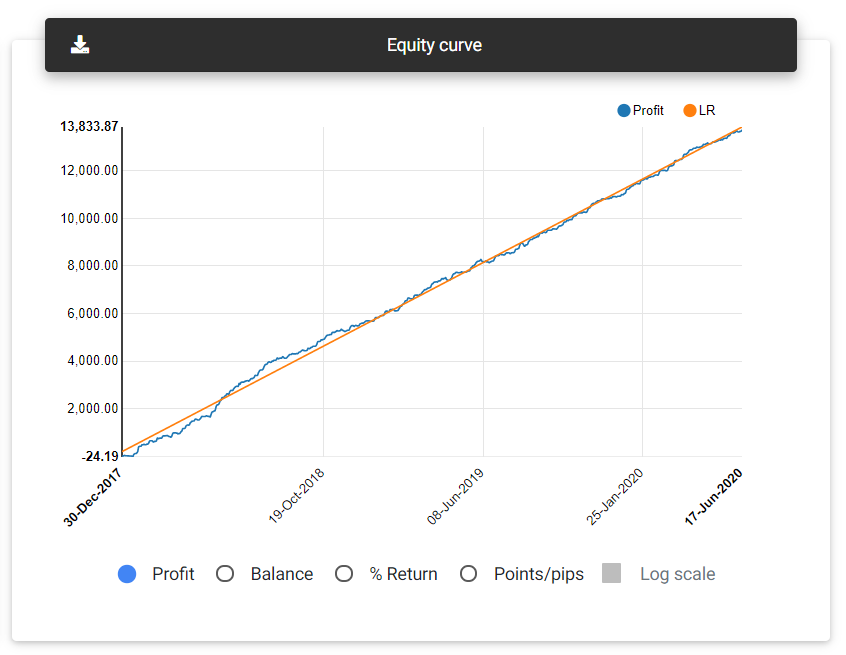Equity curve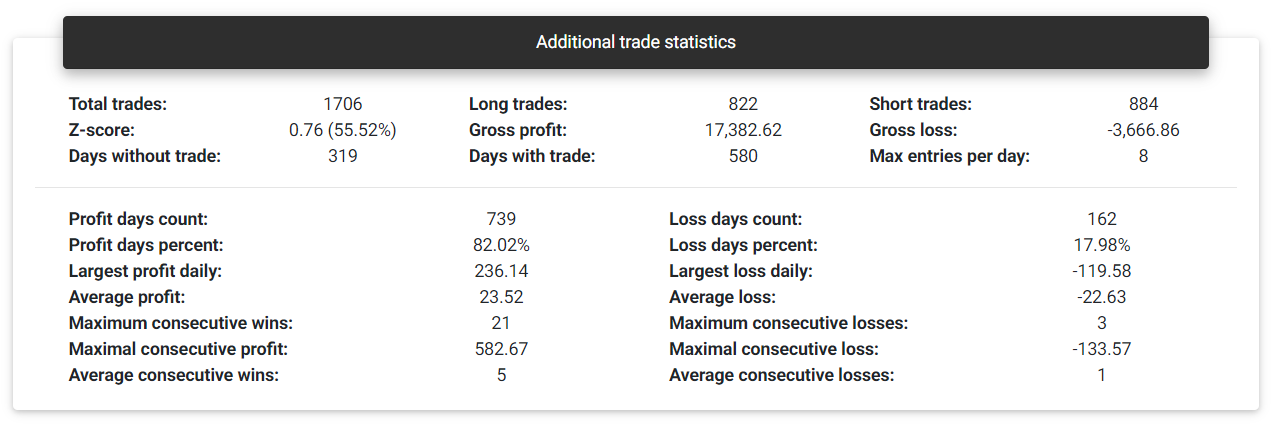Total trades: This is the total number of trades observed in the history. The app counts each closed transaction as an individual trade. If there are partial closes, each one will be considered a different trade.

Gross profit: This is the sum of all trades that ended with a profit.

Gross loss: This is the sum of all trades that ended with a loss.

Z-score: With the Z-score, it is possible to assess whether the portfolio is likely to present sequences of days with gains or losses, or whether the results will alternate. Negative Z-score values from -2.0 indicate a 95% probability that a profitable day will follow another profitable day, and likewise, a losing day will follow another losing day. Positive values starting from 2.0 suggest that with a 95% probability, a profitable day will follow a losing day and vice versa. Z-score values between -2.0 and +2.0 don't lead to a conclusion regarding the dependency.

Days without trade: Number of days that there was no trade with this portfolio.

Days with trade: Number of days that there was trade with this portfolio.

Max entries per day: Maximum number of trades in a single day.

Profit days count: Number of days that ended with positive or zero results.

Profit days percent: Percentage of days that ended with positive or zero results.

Loss days count: Number of days that ended with negative results.

Loss days percent: Percentage of days that ended with negative results.

Largest profit daily: Highest profit achieved in a single day.

Largest loss daily: Greatest loss achieved in a single day.

Average profit: Average daily result with profit.

Average loss: Average daily result with loss.

Maximum consecutive wins: Maximum number of consecutive days with profit.

Maximum consecutive losses: Maximum number of consecutive days with loss.

Maximal consecutive profit: Highest profit achieved in consecutive days.

Maximal consecutive loss: Highest loss achieved in consecutive days.

Average consecutive wins: Average number of consecutive profitable days.

Average consecutive losses: Average number of consecutive losing days.

### Return analysis

##### Monthly returns

This card shows a bar chart with the portfolio returns for each month available in history. You can see the results in percentage return, financial values, or points/pips. It's possible to select a specific year at the bottom of the chart to check the results.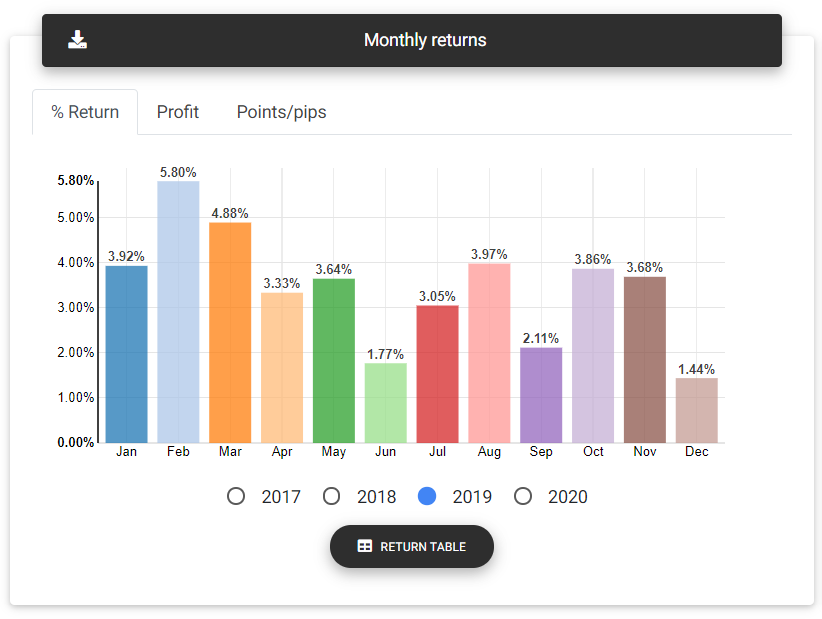Monthly returns

##### Yearly returns

This card shows the accumulated result in each year in which the trades took place. You can see the results in percentage return, financial values, or points/pips.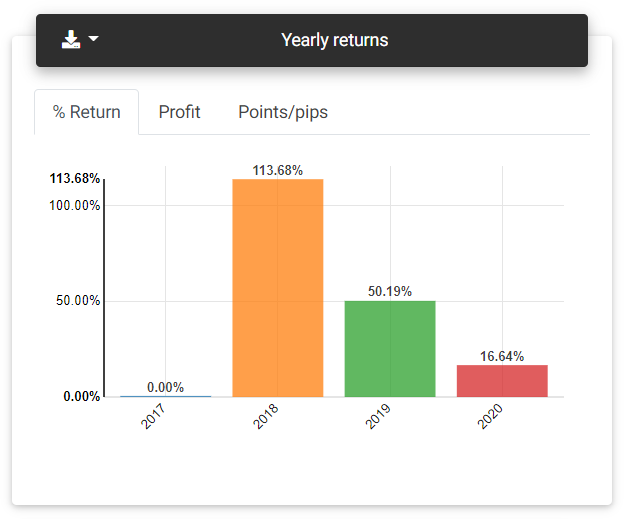Yearly returns

##### Latest returns

From the charts presented in this card, you can see the portfolio's recent results, referring to the day, week, and month, compared with the previous period.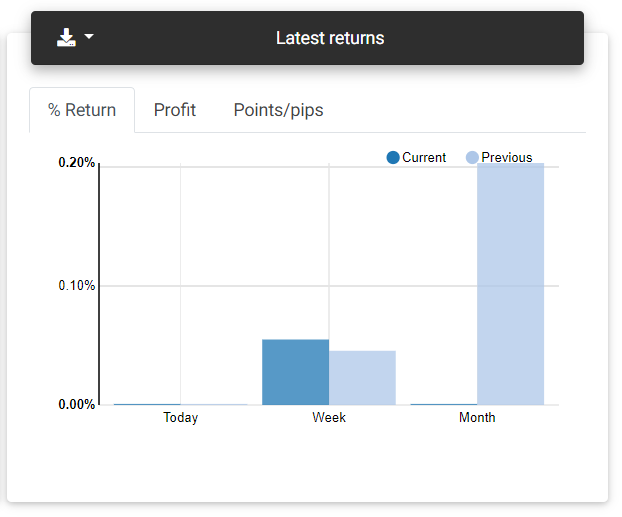Latest returns

##### Daily returns

On this card, you find a table with the returns obtained each day, ending on the last day there was a trade on the history.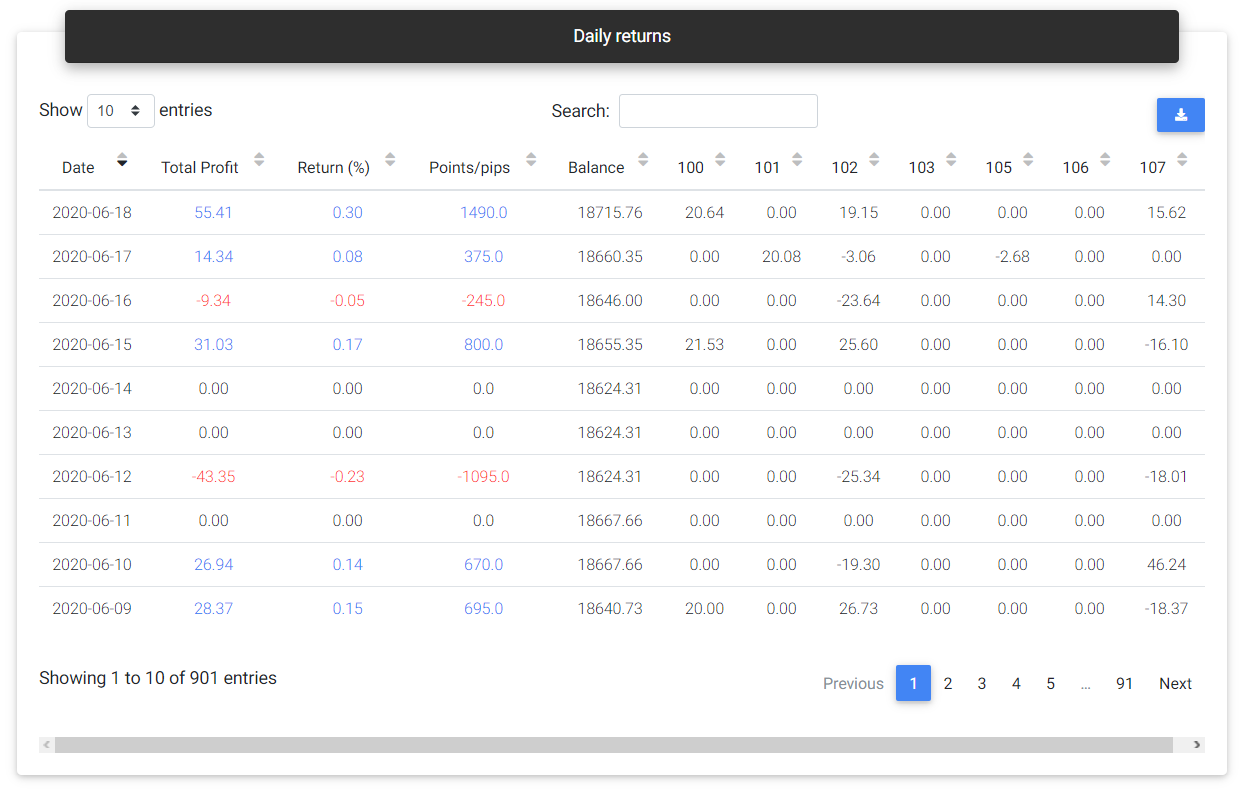Daily returns

##### Return statistics

Today: Percentage return obtained on the current day.

This week: Percentage return achieved in the current week.

This month: Percentage return achieved in the current month.

This year: Percentage return achieved in the current year.

Best year: Highest annual percentage return ever achieved by the portfolio.

Worst year: Lowest annual percentage return ever achieved by the portfolio.

Best month: Highest monthly percentage return ever achieved by the portfolio.

Worst month: Lowest monthly percentage return ever achieved by the portfolio.

Monthly Sharpe: Sharpe ratio calculated monthly.

### Drawdown and risk

##### Risk of ruin

The risk of ruin considers past results to calculate the probability of losing some part or even the total capital invested in the portfolio. Ideally, the portfolio should have a higher probability of losing smaller portions of the capital and reducing probability as a greater loss is simulated. With a chart's aid, we expect to see a curve with a decline in the risk of ruin to something near zero as the loss is close to the entire capital.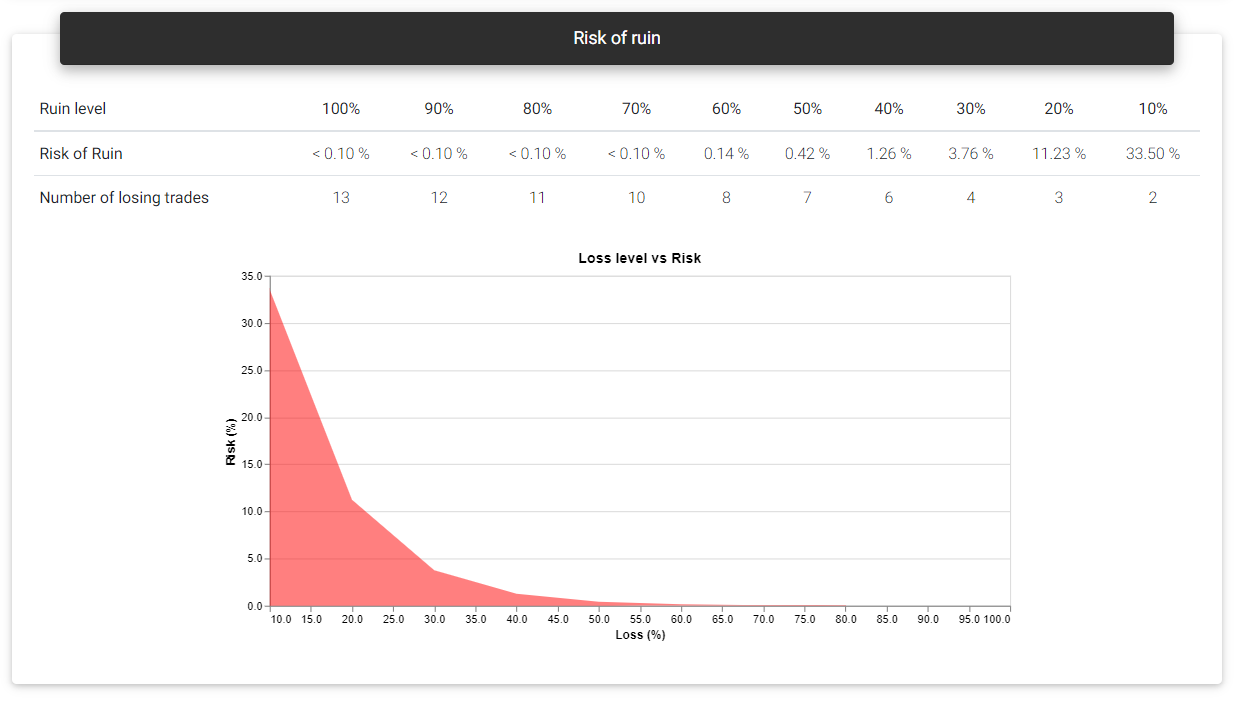Risk of ruin

##### Drawdown statistics

Maximum relative: This is the highest percentage drawdown verified in history. The percentage drawdown tends to be higher in the first trades if the historical data contains fixed lot trades. In brackets, you see the financial value corresponding to this drawdown.

Maximum absolute: Largest financial drawdown value verified. In brackets, it's shown the percentage change corresponding to this drawdown.

From initial balance: The largest financial drawdown is applied to the initial balance to give you an idea of how much this value represents in percentage terms if you faced the drawdown in the first days.

Maximum duration: Shows the length of the longest drawdown measured in days.

Average duration: This is the average length of a drawdown measured in days.

Recovery factor: This is the total net profit divided by the maximum drawdown verified. This number gives an idea of the portfolio's recovering power from drawdown periods.

##### Longest drawdowns

Drawdowns are common to all trading systems. Strategies and portfolios spend most of their time on some drawdown, as any negative trade will start or continue such a move. What you should note is the duration and size of these drawdowns, which can represent a considerable loss of capital for a long time. For example, if a portfolio takes a year to recover from a drawdown, it may not worth putting it into a real account. Perhaps you will need to make some changes to the composition. On this card, a chart gives you a general idea of ​​the drawdown periods faced by the portfolio. The primary curve is profit growth, with the five most extensive drawdown periods indicated in different colors. Also, below the profit chart, three curves representing the same drawdown levels are plotted, one in points (volume independent), another with financial value, and the percentage drawdown. These curves will appear in red whenever the portfolio remains in a drawdown period, and by hovering them, you can see the corresponding values.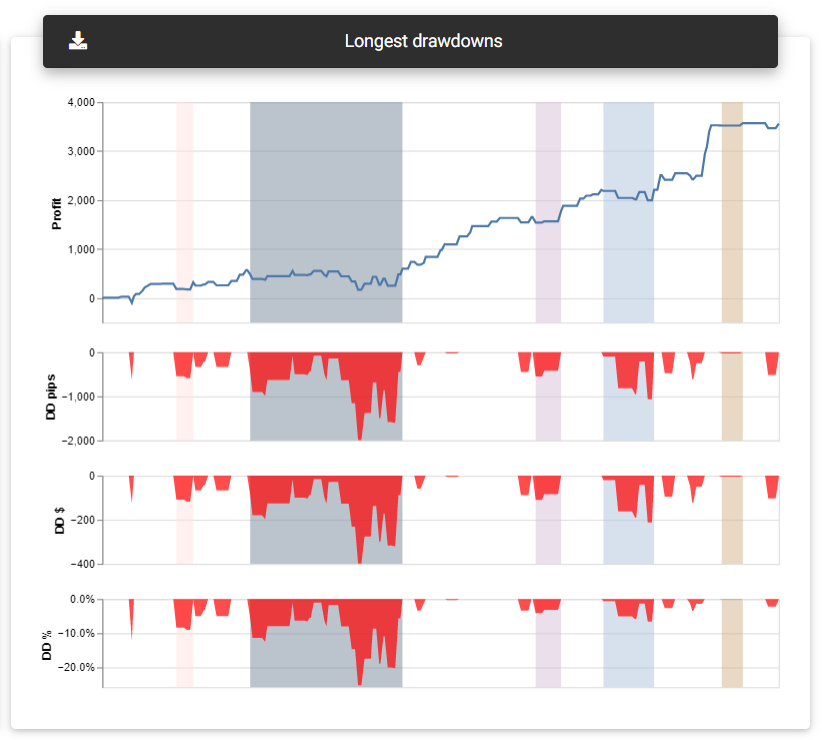Longest drawdowns

##### Drawdowns details

This table will show all the portfolio's drawdown periods, indicating the start and end dates, duration, and corresponding values in percentage, financial, and points/pips.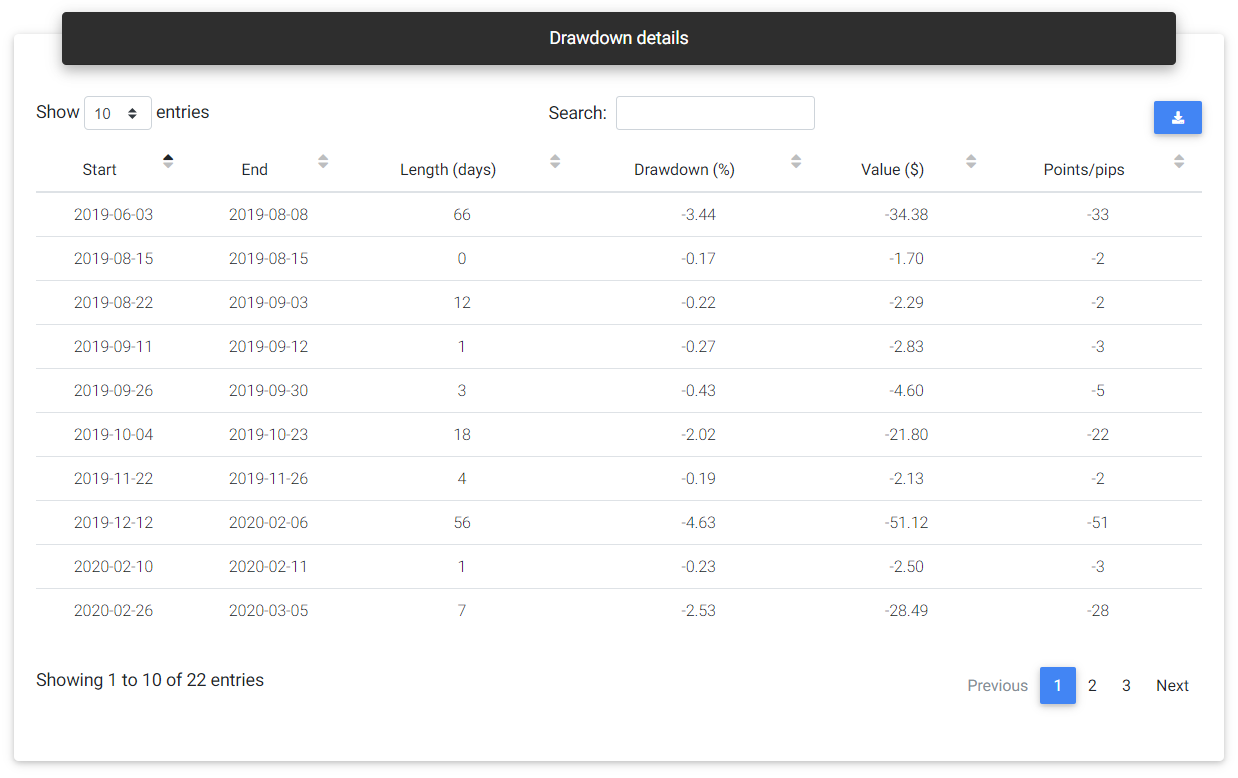Drawdown details

### Composition

Portfolios are composed of multiple strategies, and each of them can contribute differently to the final result of the portfolio. In this section, you can see some statistics related to the strategies that build up the portfolio.

##### Portfolio composition

In this frame, you can see a chart with each strategy's individual growth and the equity curve of the complete portfolio. Here it's possible to see how each strategy has performed over time and which have had a greater contribution to the portfolio's results. Highlighted, you see the best performance (highest profit) and worst-performing strategy (lowest profit).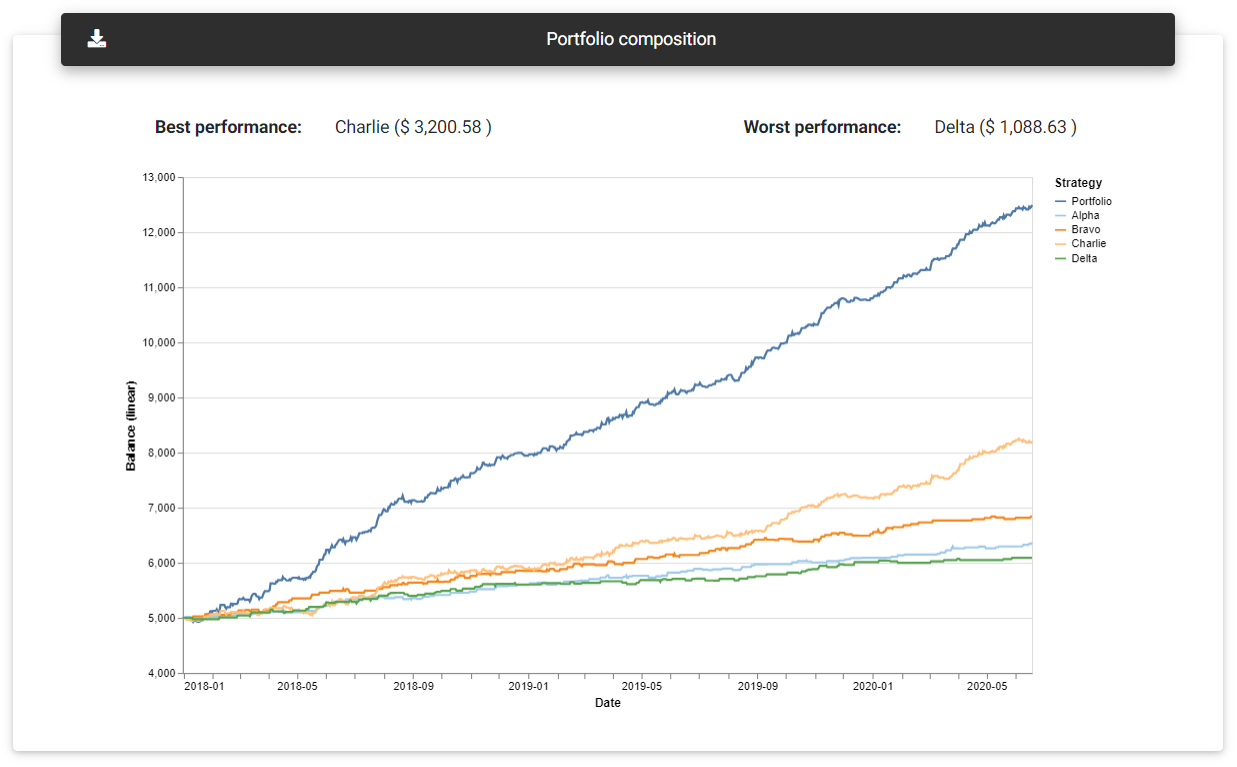Portfolio composition

##### Individual contribution

Another way to view the performance of each strategy is through the individual contribution details. Here you can see the data presented in a table, and it can be ordered in different ways to facilitate the visualization of the strategies' performance. This table shows the financial profit, profit in points/pips, percentage return, percentage drawdown, and contribution to total profit.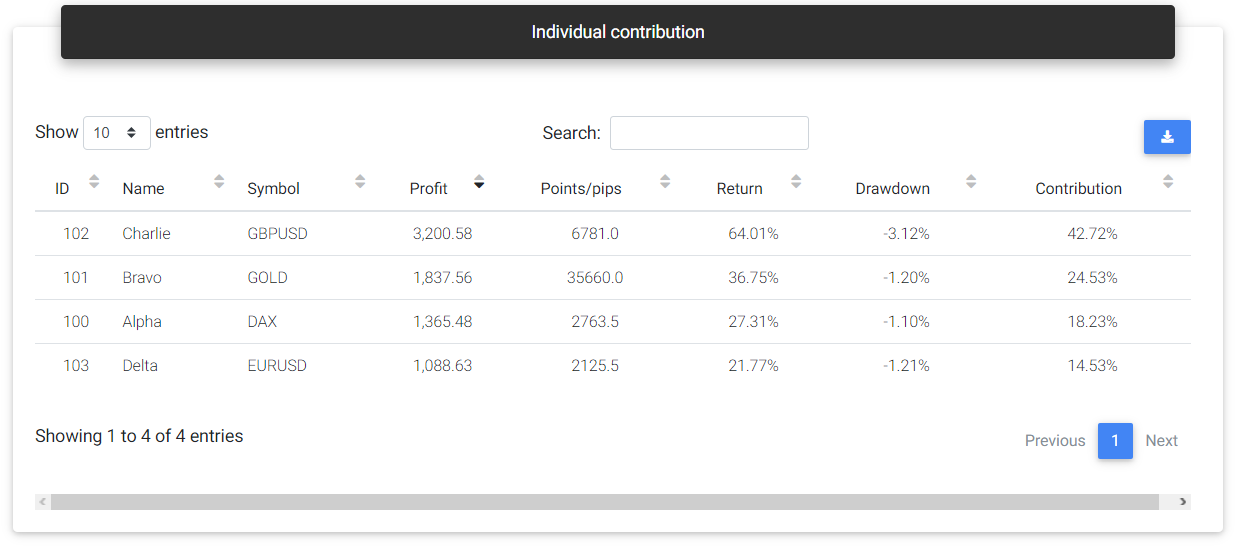Individual contribution

##### Positive strategy contribution

Among all the strategies that had a positive result, a pie chart shows each one's proportion in the total.

##### Negative strategy contribution

Among all the strategies that had a negative result, a pie chart shows each one's proportion in the total.

##### Strategy styles

This card presents the distribution of strategies' trading styles, with a pie chart representing each style percentage. In addition to the distribution of strategies by style, you can observe each style's total profit in a bar chart.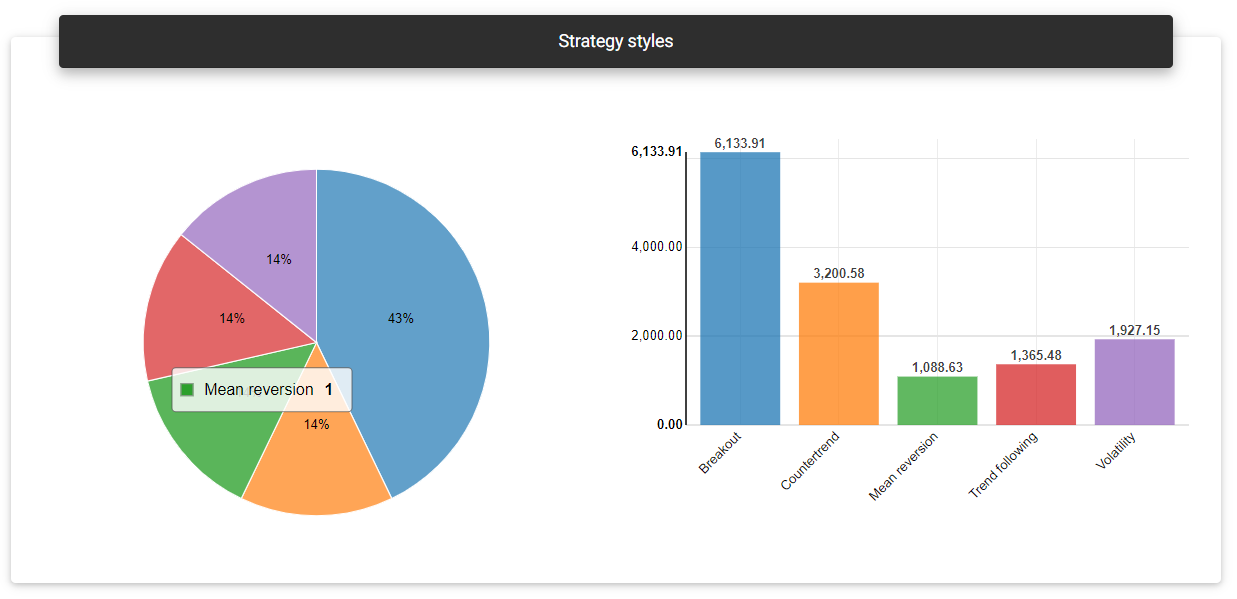Strategy styles

This card shows the distribution of strategies' trade duration, with a pie chart representing each duration percentage (day trading, swing trading, or position trading). In addition to the distribution of strategies per duration, you find each duration's total profit in a bar chart.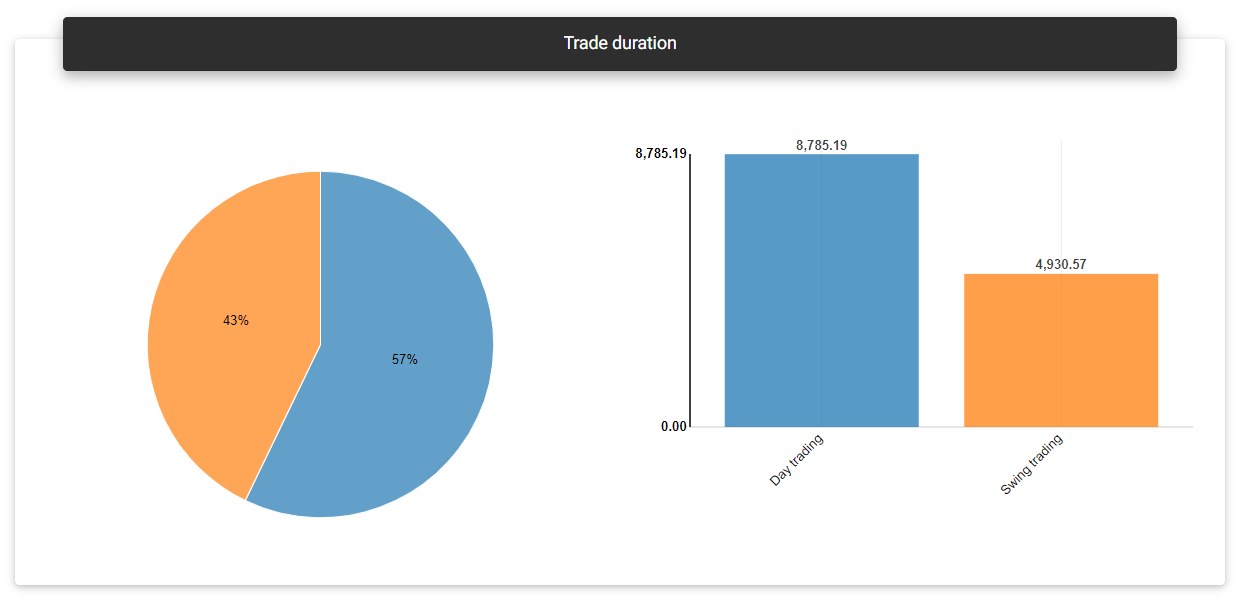##### Symbols

Here you find the symbols traded by the strategies, with a pie chart representing the symbol percentage. In addition to the distribution of strategies per symbol, a bar chart also shows each trading symbol's total profit.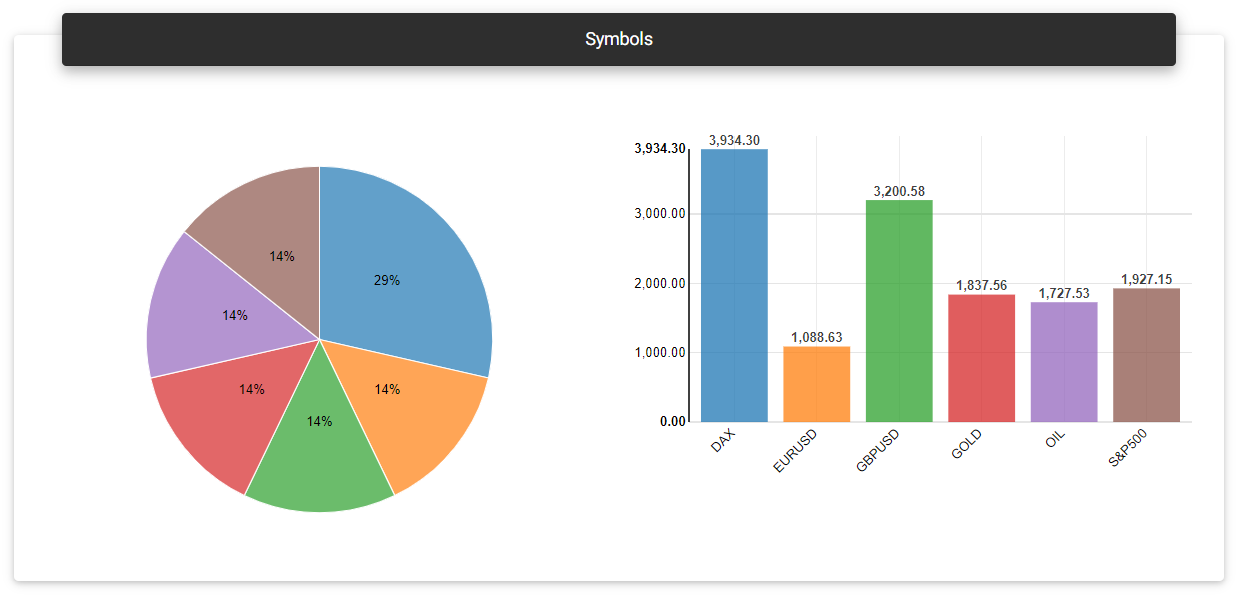Symbols

### Benchmark and forecast

##### Benchmark

On this card, you can compare your portfolio's performance with the benchmark symbol chosen, and it's also possible to choose other benchmarks for evaluation directly in the report. The app normalizes the opening balance for the two histories, and the percentage returns obtained by the benchmark and portfolio itself are applied. Low initial balances tend to overestimate the portfolios' results.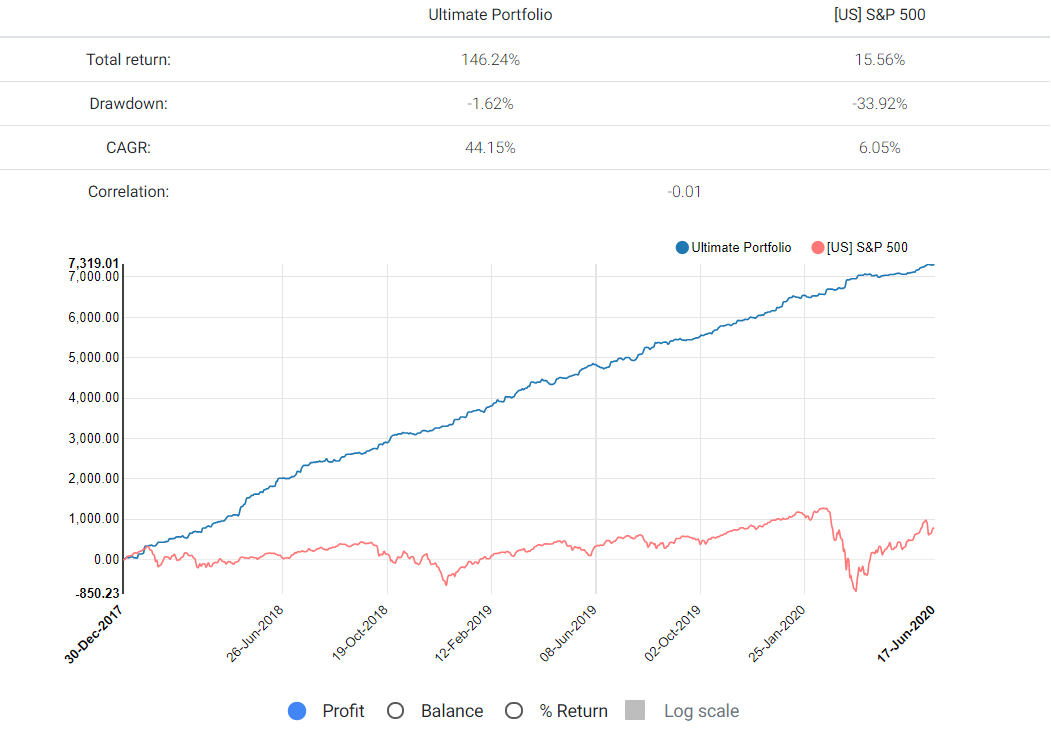Benchmark

##### Forecast

Based on the portfolio's history, the app generates a prediction model that will give a possible direction to the portfolio's balance in the following six months. The forecast line appears in green color, with the bands indicating the confidence levels or margin of error.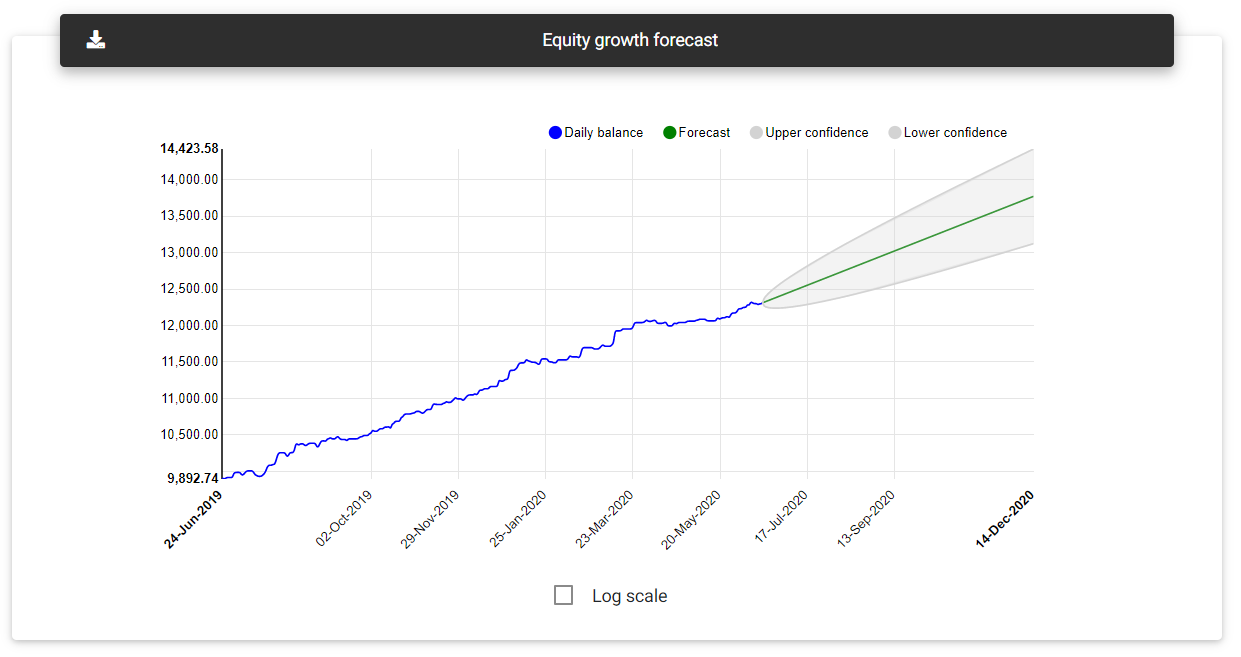Forecast